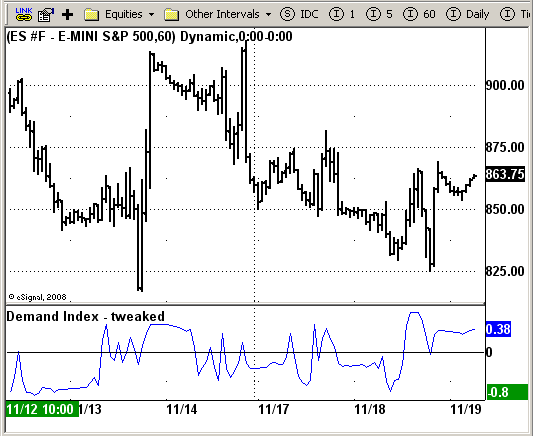# Demand Index - tweaked

ICE Data Services -

 DemandIndex.efs EFSLibrary - Discussion Board

File Name: DemandIndex.efs

Description:
Demand Index - tweaked

Formula Parameters:
Length: 5

Notes:
The Demand Index, developed by James Sibbet, combines price and volume
in such a way that it is often a leading indicator of price change.
The Demand Index calculations are too complex, however, for this text.
The calculations require 21-column accounting paper to calculate manually.`/*********************************Provided By: eSignal (Copyright c eSignal), a division of Interactive Data Corporation. 2008. All rights reserved. This sample eSignal Formula Script (EFS) is for educational purposes only and may be modified and saved under a new file name. eSignal is not responsible for the functionality once modified. eSignal reserves the right to modify and overwrite this EFS file with each new release.Description: Demand Index - tweakedVersion: 1.0 11/10/2008Formula Parameters: Default: Length 5Notes: The Demand Index, developed by James Sibbet, combines price and volume in such a way that it is often a leading indicator of price change. The Demand Index calculations are too complex, however, for this text. The calculations require 21-column accounting paper to calculate manually. **********************************/var fpArray = new Array();var bInit = false;function preMain(){ setStudyTitle("Demand Index - tweaked"); setCursorLabelName("Demand Index", 0); setDefaultBarFgColor(Color.blue, 0); addBand(0, PS_SOLID, 1, Color.black); var x=0; fpArray[x] = new FunctionParameter("Length", FunctionParameter.NUMBER); with(fpArray[x++]){ setLowerLimit(1); setDefault(5); }}var xTR = null;var xAvgTR = null;var nBuyPres_1 = 1;var nSellPres_1 = 1;var nSellPres = 1;var nBuyPres = 1;var nVolAvg_1 = 0;var nVolAvg = 0;var nWghtClose_1 = 0;var nWghtClose = 0;function main(Length) {var nWtCRatio = 1;var nVolRatio = 1;var nBuyP = 1;var nSellP = 1;var nSign = 1;var nConstant = 1;var nTempDI = 1;var nDMI = 1;var nState = getBarState(); if (nState == BARSTATE_ALLBARS) { if(Length == null) Length = 5; } if (nState == BARSTATE_NEWBAR) { nBuyPres_1 = nBuyPres; nSellPres_1 = nSellPres; nVolAvg_1 = nVolAvg; nWghtClose_1 = nWghtClose; } if ( bInit == false ) { xTR = efsInternal("TrueRange"); xAvgTR = sma(Length, xTR); bInit = true; } if (getCurrentBarCount() < Length) return; nWghtClose = (high(0) + low(0) + 2 * close(0)) * 0.25; nVolAvg = ((nVolAvg_1 * (Length - 1)) + volume(0)) / Length; if (nWghtClose != 0 && nWghtClose_1 != 0) { nWtCRatio = (nWghtClose - nWghtClose_1) / Math.min(nWghtClose, nWghtClose_1); nVolRatio = volume(0) / nVolAvg; nConstant = ((nWghtClose * 3) / xAvgTR.getValue(0)) * Math.abs(nWtCRatio); if(nConstant > 88) nConstant = 88; nConstant = nVolRatio / Math.exp(nConstant); if(nWtCRatio > 0){ nBuyP = nVolRatio; nSellP = nConstant; } else { nBuyP = nConstant; nSellP = nVolRatio; } nBuyPres = ((nBuyPres_1 * (Length - 1)) + nBuyP) / Length; nSellPres = ((nSellPres_1 * (Length - 1)) + nSellP) / Length; nTempDI = +1; if(nSellPres > nBuyPres){ nSign = -1; if(nSellPres != 0) nTempDI = nBuyPres / nSellPres; } else { nSign = +1; if(nBuyPres != 0) nTempDI = nSellPres / nBuyPres; } nTempDI = nTempDI * nSign; if(nTempDI < 0) nDMI = -1 - nTempDI; else nDMI = +1 - nTempDI; } return nDMI; }function TrueRange(){ return Math.max((high(0)-low(0)),Math.abs(close(-1)-high(0)),Math.abs(close(-1)-low(0)));} `